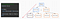# Dynamic Programming: Memoization (The Top-Down Approach)

Last week I talked about Recursion and the Fibonacci Sequence. This week I’m going to go into using a dynamic programming technique called memoization.

When we did the recursive approach we noticed a lot of the same subproblems we solved were solved multiple times which isn’t very efficient.

Using memoization, we will store the answers to subproblems that have been solved into an array.

## Recursive code vs memoization

Using the memoization approach, we will store our solved subproblems in an array (@memo). Similar to our base case in the recursive approach, if @memo[n] (0 and 1) exists then return @memo[n]. If not @memo[n] = fib(n-1) + fib(n-2).

Just like the recursive example, we’ll find out the 4th number in the Fibonacci sequence fib(4).

## Let’s break it down

Using fib(4) as our example, @memo doesn’t exist, so @memo = fib(n-1) + fib(n-2) which is fib(3) + fib(2).

@memo doesn’t exist, so @memo = fib(2) + fib(1)

@memo doesn’t exist so @memo = fib(1) + fib(0)

When we reach fib(1) @memo exists, which is 1, so we return 1. The same goes for fib(0). Therefore, @memo = 1.

So we now have @memo in our array of solved subproblems. (@memo = 1)as we can see all the boxes in red at this point are stored in our array!

Looking at the image above, we can see the entire right side of the tree has been stored in our subproblem answer array at this point! If you remember in the recursive method, each of these boxes would have to be solved but using memoization, they already are.

fib(3) is equal to @memo + @memo. @memo exists in our array so we don’t need to solve it anymore! Therefore fib(3) = 2. (@memo = 1 and @memo = 1. 1 + 1 = 2)

At this point we know fib(4) = 2 + fib(2). fib(2) (@memo) exists in our array which is @memo = 1. Therefore fib(4) = 2 + 1 which is 3. The 4th position in the Fibonacci Sequence is 3. (1 1 2 3).

We started from the top, worked our way down to our base case. Next week I’ll go over the bottom-up iterative approach.

Fullstack Software Engineer

## More from Alex Duterte

Fullstack Software Engineer